# Certain and Impossible Events

Experiment 1: A spinner has 4 equal sectors colored yellow, blue, green, and red. What is the probability of landing on purple after spinning the spinner?Probability: It is impossible to land on purple since the spinner does not contain this color.

 P(purple) = 0 = 0 4Experiment 2: A teacher chooses a student at random from a class of 30 girls. What is the probability that the student chosen is a girl?

Probability: Since all the students in the class are girls, the teacher is certain to choose a girl.

 P(girl) = 30 = 1 30

In the first experiment, it was not possible to land on purple. This is an example of an impossible event. In the second experiment, choosing a girl was certain to occur. This is an example of a certain event.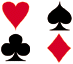The next experiment will involve a standard deck of 52 playing cards, which consists of 4 suits: hearts, clubs, diamonds and spades. Each suit has 13 cards as follows: ace, deuce, three, four, five, six, seven, eight, nine, ten, jack, queen, and king. Picture cards include jacks, queens and kings. There are no joker cards. There are only 4 of a kind, for example, 4 tens.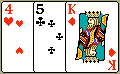Experiment 3: A single card is chosen at random from a standard deck of 52 playing cards. What is the probability that the card chosen is a joker card?

Probability: It is impossible to choose a joker card since a standard deck of cards does not contain any jokers. This is an impossible event.

 P(joker) = 0 = 0 52

Experiment 4: A single 6-sided die is rolled. What is the probability of rolling a number less than 7?

Probability: Rolling a number less than 7 is a certain event since a single die has 6 sides, numbered 1 through 6.

 P(number < 7) = 6 = 1 6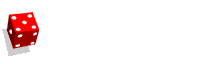Experiment 5: A total of five cards are chosen at random from a standard deck of 52 playing cards. What is the probability of choosing 5 aces?

Probability: It is impossible to choose 5 aces since a standard deck of cards has only 4 of a kind. This is an impossible event.

 P(5 aces) = 0 = 0 52Experiment 6: A glass jar contains 15 red marbles. If a single marble is chosen at random from the jar, what is the probability that it is red?

Probability: Choosing a red marble is certain to occur since all 15 marbles in the jar are red. This is a certain event.

 P(red) = 15 = 1 15

Summary: The probability of an event is the measure of the chance that the event will occur as a result of the experiment. The probability of an event A, symbolized by P(A), is a number between 0 and 1, inclusive, that measures the likelihood of an event in the following way:

• If P(A) > P(B) then event A is more likely to occur than event B.
• If P(A) = P(B) then events A and B are equally likely to occur.
• If event A is impossible, then P(A) = 0.
• If event A is certain, then P(A) = 1.

### Exercises

Directions: Read each question below. Select your answer by clicking on its button. Feedback to your answer is provided in the RESULTS BOX. If you make a mistake, choose a different button.

 1. A glass jar contains 5 red, 3 blue and 2 green jelly beans. If a jelly bean is chosen at random from the jar, then which of the following is an impossible event? Choosing a red jelly bean.Choosing a blue jelly bean.Choosing a yellow jelly bean.None of the above. RESULTS BOX:
 2. A spinner has 7 equal sectors numbered 1 to 7. If you spin the spinner, then which of the following is a certain event? Landing on a number less than 7.Landing on a number less than 8.Landing on a number greater than 1.None of the above. RESULTS BOX:
 3. What is the probability of choosing 14 hearts from a standard deck of 52 playing cards?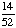1 0 None of the above. RESULTS BOX:
 4. If a number is chosen at random from the following list, then what is the probability that it is prime? 2, 3, 5, 7, 11, 13, 17, 19 1  0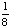None of the above. RESULTS BOX:
 5. If a single 6-sided die is rolled, then which of the following events is neither certain nor impossible? Rolling a number less than 7.Rolling an even number.Rolling a zero.None of the above. RESULTS BOX: